# 6th-8th Grade Geometry: Triangles Chapter Exam

Exam Instructions:

Choose your answers to the questions and click 'Next' to see the next set of questions. You can skip questions if you would like and come back to them later with the yellow "Go To First Skipped Question" button. When you have completed the practice exam, a green submit button will appear. Click it to see your results. Good luck!

### Page 1

#### Question 1 1. What type of line segment is represented by the vertical dotted line in the pictured triangle?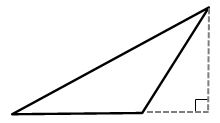#### Question 3 3. If angle a is 68 degrees and angle b is 37 degrees, what is the measure of angle c?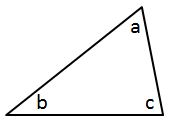### Page 2

#### Question 8 8. In the figure below, Angle 7 = 15x and Angle 10 = 9x + 30. Determine the measure of Angle 10.#### Question 9 9. In triangle GBN, Angle G is seven more than three times a number, Angle B is five more than four times the number, and Angle N is twelve less than five times the number. Solve for the measure of Angle G.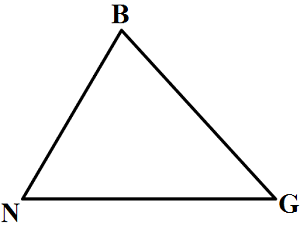#### Question 10 10. Mary claims that the triangles below are similar by AA. Do you agree or disagree with Mary?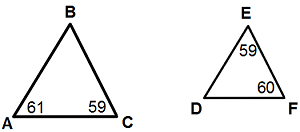### Page 3

#### Question 11 11. In the figure below, Angle 13 = 10y and Angle 11 = 3y + 11. Determine the measure of Angle 16.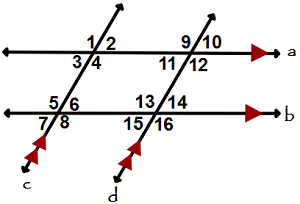#### Question 13 13. In the pictured triangle, which of the following lines is an altitude?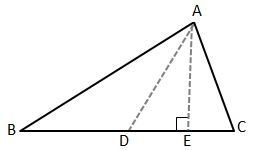#### Question 14 14. What type of triangle is pictured?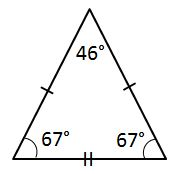#### Question 15 15. If angle a is 60 degrees, what is the measure of angle b?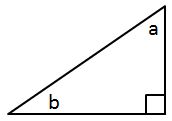### Page 4

#### Question 16 16. What type of line segment is represented by the dotted line in the pictured triangle?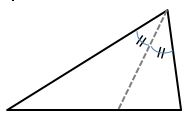#### Question 17 17. What type of triangle is pictured?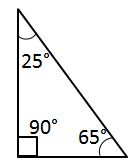#### Question 18 18. Given the triangle PQE, calculate the measure of Angle Q.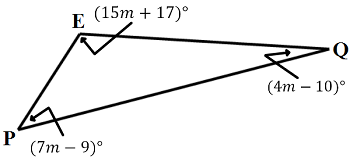#### Question 19 19. Which statement is true regarding areas based on the pictured triangle? Point G is the centroid.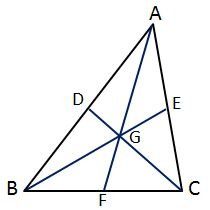### Page 5

#### Question 22 22. What type of triangle is pictured?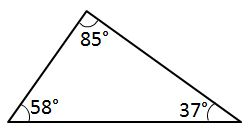#### Question 24 24. Which statement is true about the pictured triangle?#### Question 25 25. If angle a is 30 degrees and angle d is 70 degrees, what is the measure of angle b?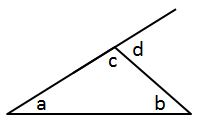### Page 6

#### Question 27 27. In the pictured triangle, what is the centroid?#### Question 28 28. Which statement is true regarding areas based on the pictured triangle? Point G is the centroid.#### Question 29 29. Given the triangle ABC, solve for the value of y.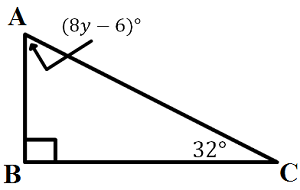#### Question 30 30. In the pictured triangle, what is side A, relative to angle x?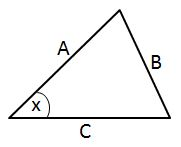#### 6th-8th Grade Geometry: Triangles Chapter Exam Instructions

Choose your answers to the questions and click 'Next' to see the next set of questions. You can skip questions if you would like and come back to them later with the yellow "Go To First Skipped Question" button. When you have completed the practice exam, a green submit button will appear. Click it to see your results. Good luck!

Support